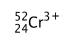# IMAT 2016 Q50 [Ions]

Identify the number of protons (p), neutrons (n) and electrons (e) present in the ion:A. p = 27 n = 25 e = 21
B. p = 24 n = 28 e = 24
C. p = 27 n = 25 e = 24
D. p = 24 n = 28 e = 21
E. p = 24 n = 24 e = 21